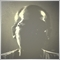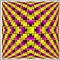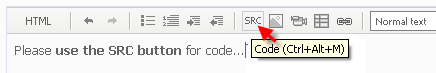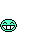# How do I write a script of lots in the EA to comply with the rules of the ATC 2012?6023

How do I write a script of lots in the EA to comply with the rules of the ATC 2012. That rule no IV.6 and IV.6.
IV.4. The minimum trading amount is 0:01 lots, and the maximum is 5 lots, with an increase of by 0.01 lot on every order.
IV.6. The maximum allowed total volume of an open positions and pending orders in one direction (either buy or sell) is 15 lots for each symbol. That it means if you have an 15-lot open Buy position, you can place a 15-lot sell limit orders pending. But in this case you can not place a Buy Limit pending order (since the total volume in one direction will exceed the limit) or place a sell limit order above 15 lots.
Thus Spake, it is theoretically possible to have open positions with the total volume up to 180 lots: a 15-lot position for each of the 12 symbols (12 * 15 = 180). Stop Loss and Take Profit orders are not taken into account.
Documentation on MQL5: Standard Constants, Enumerations and Structures / Trade Constants / Order Properties
• www.mql5.com
Standard Constants, Enumerations and Structures / Trade Constants / Order Properties - Documentation on MQL51863

achidayat:
How do I write a script of lots in the EA to comply with the rules of the ATC 2012. That rule no IV.6 and IV.6.
IV.4. The minimum trading amount is 0:01 lots, and the maximum is 5 lots, with an increase of by 0.01 lot on every order.
IV.6. The maximum allowed total volume of an open positions and pending orders in one direction (either buy or sell) is 15 lots for each symbol. That it means if you have an 15-lot open Buy position, you can place a 15-lot sell limit orders pending. But in this case you can not place a Buy Limit pending order (since the total volume in one direction will exceed the limit) or place a sell limit order above 15 lots.
Thus Spake, it is theoretically possible to have open positions with the total volume up to 180 lots: a 15-lot position for each of the 12 symbols (12 * 15 = 180). Stop Loss and Take Profit orders are not taken into account.

Hi achidayat,

Use SymbolInfoDouble () (<<== click it) using ENUM_SYMBOL_INFO DOUBLE  (<<== click again) SYMBOL_VOLUME_MIN, SYMBOL_VOLUME_STEP, SYMBOL_VOLUME_MAX, and SYMBOL_VOLUME_LIMIT (<<== search for them and please read the explanation).

:D6023

onewithzachy:

Hi achidayat,

Use SymbolInfoDouble () (<<== click it) using ENUM_SYMBOL_INFO DOUBLE  (<<== click again) SYMBOL_VOLUME_MIN, SYMBOL_VOLUME_STEP, SYMBOL_VOLUME_MAX, and SYMBOL_VOLUME_LIMIT (<<== search for them and please read the explanation).

:D

Thank you for your help. Could you give example of this script? For example GetLots() function.1863

achidayat:
Thank you for your help. Could you give example of this script? For example GetLots() function.

Hi achidayat,

:D

Documentation on MQL5: Trade Functions / OrderGetTicket
• www.mql5.com
Trade Functions / OrderGetTicket - Documentation on MQL56023

onewithzachy:

Hi achidayat,

:D

double GetLots() {
double lots;
if (MM) lots=AccountInfoDouble(ACCOUNT_FREEMARGIN)*Risk/100000; else lots=Lots;
lots=MathMin(15,MathMax(0.01,lots));
if (lots>5) lots=5;
if(lots<0.1)
lots=NormalizeDouble(lots,2);
else {
if(lots<1) lots=NormalizeDouble(lots,1);
else       lots=NormalizeDouble(lots,0);
}
return(lots);

}1863

Hi achidayat,

I hope you don't mind waiting, I kinda busy and this is after all a weekend. I already write some part of the code but haven't check throughoutly.

By the way use SRC button to post your codes.

:DHere's the codes. compiled no error just warning. Since this is weekend I can not test it - for example the value of  "Margin_Init     = SymbolInfoDouble(Symbol(),SYMBOL_MARGIN_INITIAL);" is zero, it should not zero, but we'll see on Monday.

Study it first, if you don't understand, ask a question by telling which part that you don't understand.

I welcome other forumer for their constructive opinions and comments.

:D

[Edit : Originally posted on Saturday June 30th, edited this Monday July 2nd. My apology for any inconvenience :).]

```//--- global variables
input double Lot_Size=0.01; //
input double Stop_Loss=0.0;
//+------------------------------------------------------------------+
//|                                                                  |
//+------------------------------------------------------------------+
double Lot_Calculation (int type) //--- 1 for long and -1 for short
{
double Lot, Min_Lot, Step_Lot, Max_Lot, Aggregate_Lot, Account_Balance, Margin_Init,
Total_Open_Volume, Remaining_Volume;
uint  total;
int size_step;
string text1;

//--- 1 ---
if (type == 0)
{
Print("Can not calculate lot size. Wrong direction for position opening.");
return (0);
}

//--- 2 ---
Account_Balance = AccountInfoDouble(ACCOUNT_BALANCE);
Margin_Init     = SymbolInfoDouble(Symbol(),SYMBOL_MARGIN_INITIAL);
Min_Lot         = SymbolInfoDouble(Symbol(),SYMBOL_VOLUME_MIN);
Step_Lot        = SymbolInfoDouble(Symbol(),SYMBOL_VOLUME_STEP);
Max_Lot         = SymbolInfoDouble(Symbol(),SYMBOL_VOLUME_MAX);
Aggregate_Lot   = SymbolInfoDouble(Symbol(),SYMBOL_VOLUME_LIMIT);

//--- 3A ---
Total_Open_Volume = 0.0; Remaining_Volume = 0.0;

//--- 3B ---
total = PositionsTotal();
for (uint i = 0;i < total; i++)
{
if (PositionGetSymbol(i) == Symbol())
{
switch (PositionGetInteger (POSITION_TYPE))
{
{
if (type > 0)
{
Total_Open_Volume += PositionGetDouble(POSITION_VOLUME);
text1 = "long";
break;
}
}
case POSITION_TYPE_SELL :
{
if (type < 0)
{
Total_Open_Volume += PositionGetDouble(POSITION_VOLUME);
text1 = "short";
break;
}
}
}
}
}

//--- 3C ---
total = OrdersTotal();
for (uint i = 0;i < total; i++)
{
if (OrderGetTicket(i) > 0 && OrderGetString(ORDER_SYMBOL) == Symbol())
{
switch (OrderGetInteger (ORDER_TYPE))
{
{
if (type > 0)
{
Total_Open_Volume += OrderGetDouble(ORDER_VOLUME_CURRENT);
text1 = "long";
}
break;
}
//case ORDER_TYPE_SELL :
case ORDER_TYPE_SELL_LIMIT :
case ORDER_TYPE_SELL_STOP :
case ORDER_TYPE_SELL_STOP_LIMIT :
{
if (type < 0)
{
Total_Open_Volume += OrderGetDouble(ORDER_VOLUME_CURRENT);
text1 = "short";
}
break;
}
}
}
}

//--- 4 --- the remaining volume is bigger or equal that the aggregate
if (Aggregate_Lot > 0 && (Remaining_Volume = Aggregate_Lot - Total_Open_Volume) <= 0)
{
Print ("Total volume for ",text1," has reach the limit of aggregate volume.");
return (0);
}

//--- 5 ---
if (Lot_Size >= 0)                                 //--- lot size is fixed by user
{
Lot = Lot_Size;
}
else                                            //--- lot size is percentage of account balance
{
Lot = -Lot_Size;
Money_Use_To_Trade = Lot/100 * Account_Balance; //--- this is the ammount of money to open position

Value_Per_Point = Tick_Value / Tick_Size;       //--- calculating loss value
SL = Stop_Loss;
if (SL  <= 0) SL = 100;
Loss_By_SL = SL * Value_Per_Point;

Lot = NormalizeDouble(Money_Use_To_Trade / (Loss_By_SL + Margin_Init), 2); //--- the resulting lot
}

//--- 6 ---
if (Lot > Remaining_Volume && Aggregate_Lot > 0) Lot = Remaining_Volume;  //--- lot size is bigger that available allowed

//--- 7 ---
if (Lot < Min_Lot)                                   //--- lot size is smaller than minimum required lot
{
Print ("You can\'t afford to open even the smallest lot.");
return(0);
}
else                                               //--- calculating lot size using required lot step.
{
Lot -= Min_Lot;
if (Lot >= Step_Lot) size_step = Lot / Step_Lot;
Lot = Min_Lot + (size_step* Step_Lot);
}

if (Lot > Max_Lot)Lot = Max_Lot;                      //--- lot size is bigger than maximum alowed lot

return (Lot);
}
//+------------------------------------------------------------------+
//|                                                                  |
//+------------------------------------------------------------------+```

:D6023

```double GetLots() {
double lots;
if (MM) lots=AccountInfoDouble(ACCOUNT_FREEMARGIN)*Risk/100000; else lots=Lots;
lots=MathMin(15,MathMax(0.01,lots));
if (lots>5) lots=5;
if(lots<0.1)
lots=NormalizeDouble(lots,2);
else {
if(lots<1) lots=NormalizeDouble(lots,1);
else       lots=NormalizeDouble(lots,0);
}
return(lots);

}```6023

I want to the point. How to aplly this lot function to multicurrency EA in this link https://www.mql5.com/en/code/450 and one more question why Stop_Loss including to this function?
Exp_TEMA
• 2011.09.29
• Nikolay Kositsin | English Russian Chinese Spanish Portuguese
• www.mql5.com
Multi-currency trend following expert system using the Triple Exponential Moving Average technical indicator.1863

achidayat:

I want to the point. How to aplly this lot function to multicurrency EA in this link https://www.mql5.com/en/code/450 and one more question why Stop_Loss including to this function?

Hi achidayat,

1. If your account balance is \$100,000.- and you risk 10% - that is you risk \$ 1,000 - when your stop loss is hit you only loss \$ 1,000, that is why Stop_Loss is included.

2. Find lot or volume calculation in the EA and replace it, test it on ST or on Monday. Find it all by your self. You already write your own lot calculation function, so if you use it, where you're going to put it inside the EA anyway ?

:|1863

Look like the return value of

`SymbolInfoDouble(Symbol(),SYMBOL_MARGIN_INITIAL);`

is zero !?!?!.

See, if I have time to use CSymbolInfo class or even going the old fashion way. I didn't calculate the initial margin for pending orders anyway.

:(

Documentation on MQL5: Standard Constants, Enumerations and Structures / Environment State / Symbol Properties
• www.mql5.com
Standard Constants, Enumerations and Structures / Environment State / Symbol Properties - Documentation on MQL51863

onewithzachy 2012.07.02 10:01

Look like the return value of

`SymbolInfoDouble(Symbol(),SYMBOL_MARGIN_INITIAL);`

is zero !?!?!.

See, if I have time to use CSymbolInfo class or even going the old fashion way. I didn't calculate the initial margin for pending orders anyway.

:(

Hi old myself,

Here's the discussion : https://www.mql5.com/en/forum/7418 clcik that.(bug?) SYMBOL_MARGIN_INITIAL = 0.0
• www.mql5.com
"SYMBOL_MARGIN_INITIAL Initial margin means the amount in the margin currency required for opening a position with the volume of one lot.